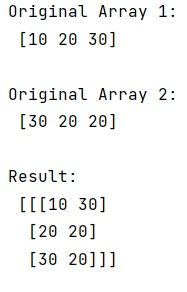# What is the numpy.dstack() function in NumPy?

Learn about the Python's numpy.dstack() function in NumPy with example.
Submitted by Pranit Sharma, on February 19, 2023

NumPy is an abbreviated form of Numerical Python. It is used for different types of scientific operations in python. Numpy is a vast library in python which is used for almost every kind of scientific or mathematical operation. It is itself an array which is a collection of various methods and functions for processing the arrays.

## numpy.dstack() function

In numpy, the dstack() method is used to stack arrays in sequence depth-wise (along the third axis).

This is equivalent to concatenation along the third axis after 2-D arrays of shape (M, N) have been reshaped to (M, N, 1) and 1-D arrays of shape (N,) have been reshaped to (1, N, 1).

It takes a parameter called tup which is the sequence of arrays. The arrays must have the same shape along all but the third axis. 1-D or 2-D arrays must have the same shape.

It returns an array formed by stacking the given arrays, the returned array will be at least a 3D array.

Let us understand with the help of an example,

## Python code to demonstrate the numpy.dstack() function in NumPy

```# Import numpy
import numpy as np

# Creating two numpy arrays
arr1 = np.array([10, 20, 30])
arr2 = np.array([30, 20, 20])

# Display original arrays
print("Original Array 1:\n",arr1,"\n")
print("Original Array 2:\n",arr2,"\n")

# Using dstack method
res = np.dstack((arr1,arr2))

# Display result
print("Result:\n",res,"\n")
```

Output: